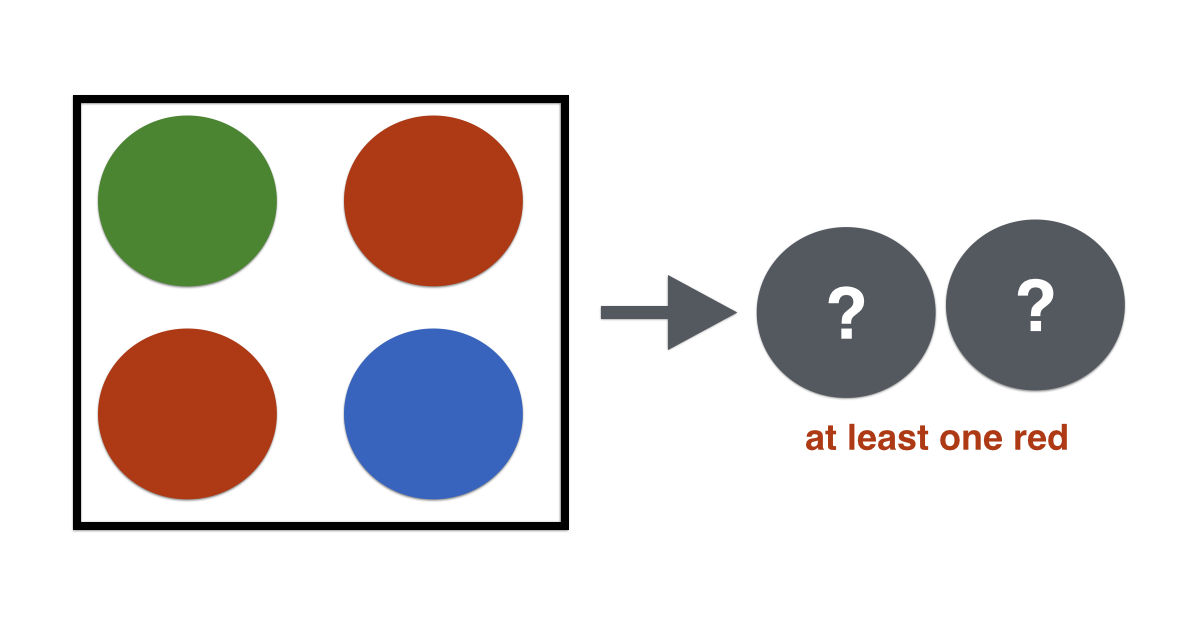Probability

# Conditional Probability: Level 4 ChallengesOne green ball, one blue ball, and two red balls are placed in a bowl. I draw two balls simultaneously from the bowl and announce that at least one of them is red.

What is the chance that the other ball I have drawn out is also red?

Box I contains 1 red ball and 2 white balls. Box II contains 2 red balls and 1 white ball. One ball is drawn randomly from box I and transferred to box II.

Then, a ball is drawn randomly from box II and it is red. What is the probability that the transferred ball was white?

There are two bags $A$ and $B$. Bag $A$ contains $n$ white balls and $2$ black balls while bag $B$ contains $2$ white balls and $n$ black balls . One of the two bags is selected at random and two balls are drawn from it without replacement . We know that if both the balls drawn are white balls, the probability of bag $A$ being selected to draw the balls is $\frac{6}{7}$. Find the value of $n$.

On planet Brilliantia, there are two types of creatures: mathematicians and non-mathematicians.

Mathematicians tell the truth $\frac{6}{7}$ of the time and lie only $\frac{1}{7}$ of the time, while non-mathematicians tell the truth $\frac{1}{5}$ of the time and lie $\frac{4}{5}$ of the time.

It is also known that there is a $\frac{2}{3}$ chance a creature from Brilliantia is a mathematician and a $\frac{1}{3}$ chance that it is a non-mathematician, but there is no way of differentiating from these two types.

You are visiting Brilliantia on a research trip. During your stay, you come across a creature who states that it has found a one line proof for Fermat's Last Theorem. Immediately after that, a second creature shows up and states that the first creature's statement was a true one.

If the probability that the first creature's statement was actually true is $\frac{a}{b}$, for some coprime positive integers $a, b$, find the value of $b - a$.

Calvin flips a fair coin before he sees a run of an odd number of heads, followed by a tail. What is the expected number of times Calvin must flip the coin?

×